#TensorFlow

0
1
01. 云栖社区>
2. 博客>
3. 正文

## TensorFlow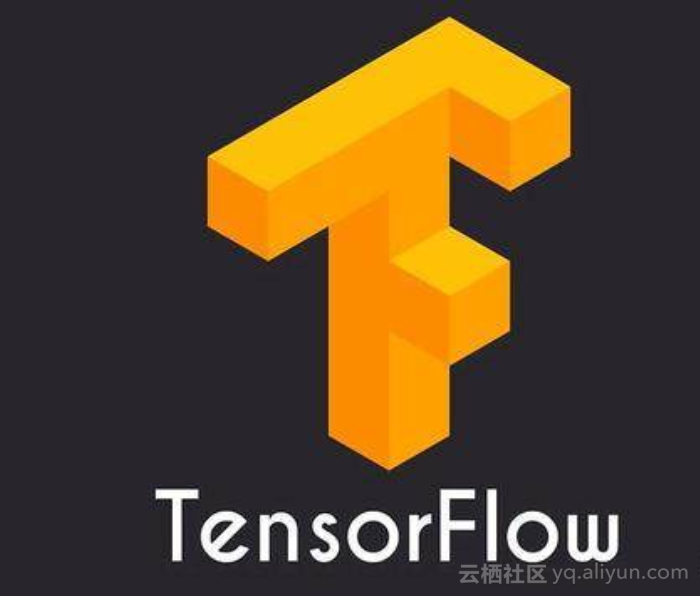TensorFlow 不是一个标准的 Python 库

Python 和 TensorFlow 之间的关系可以类比 Javascript 和 HTML 之间的关系。Javascript 是一种全功能的编程语言，可以做各种美妙的事情。HTML 是用于表示某种类型的实用计算抽象（此处指可由 Web 浏览器呈现的内容）的框架。Javascript 在交互式网页中的作用是组装浏览器看到的 HTML 对象，然后在需要时通过将其更新为新的 HTML 来与其交互。

import tensorflow as tfimport tensorflow as tftwo_node = tf.constant(2)print two_node

Tensor("Const:0", shape=(), dtype=int32)import tensorflow as tftwo_node = tf.constant(2)another_two_node = tf.constant(2)two_node = tf.constant(2)tf.constant(3)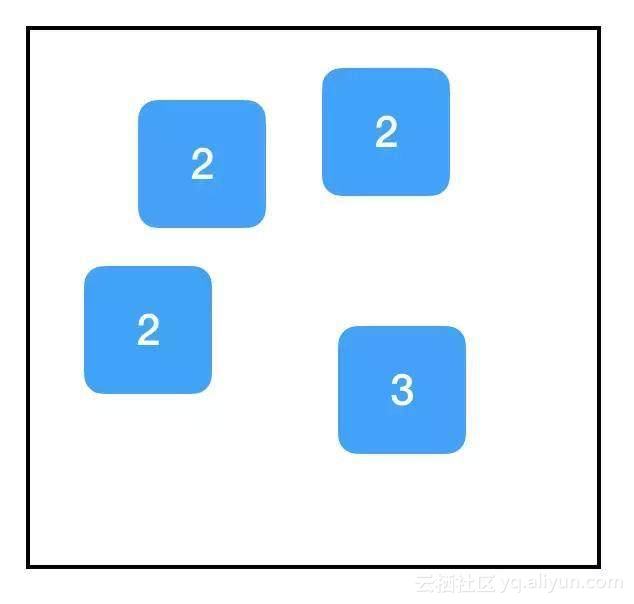import tensorflow as tftwo_node = tf.constant(2)another_pointer_at_two_node = two_nodetwo_node = Noneprint two_nodeprint another_pointer_at_two_node

NoneTensor("Const:0", shape=(), dtype=int32)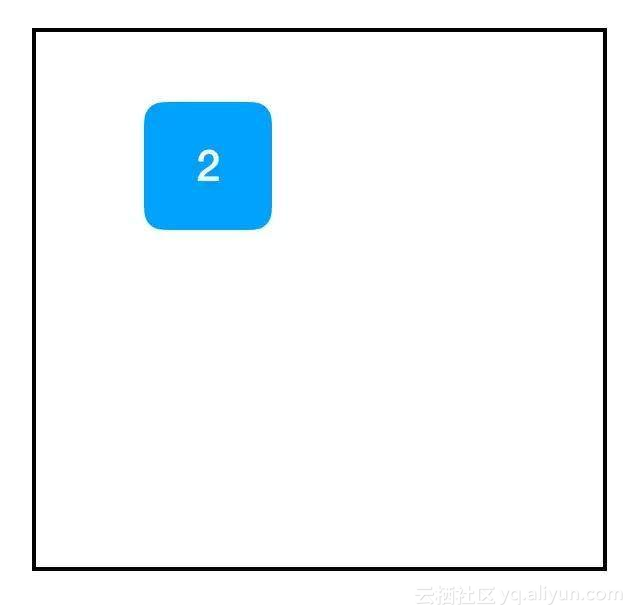import tensorflow as tftwo_node = tf.constant(2)three_node = tf.constant(3)sum_node = two_node + three_node ## equivalent to tf.add(two_node, three_node)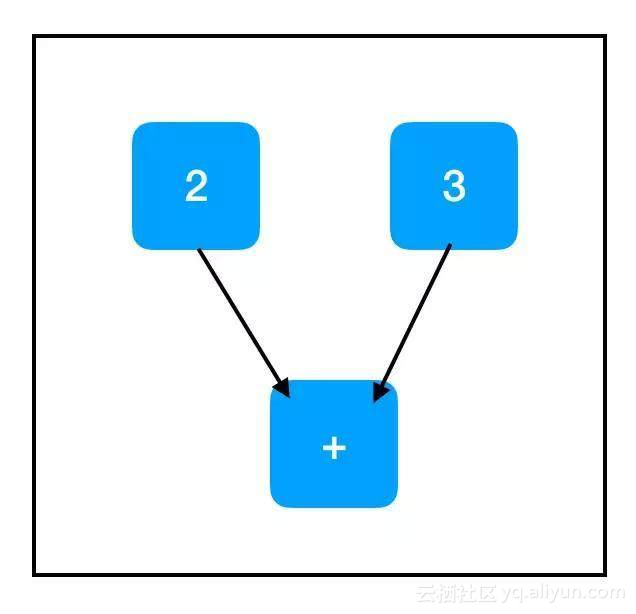import tensorflow as tftwo_node = tf.constant(2)three_node = tf.constant(3)sum_node = two_node + three_nodesess = tf.Session()print sess.run(sum_node)

5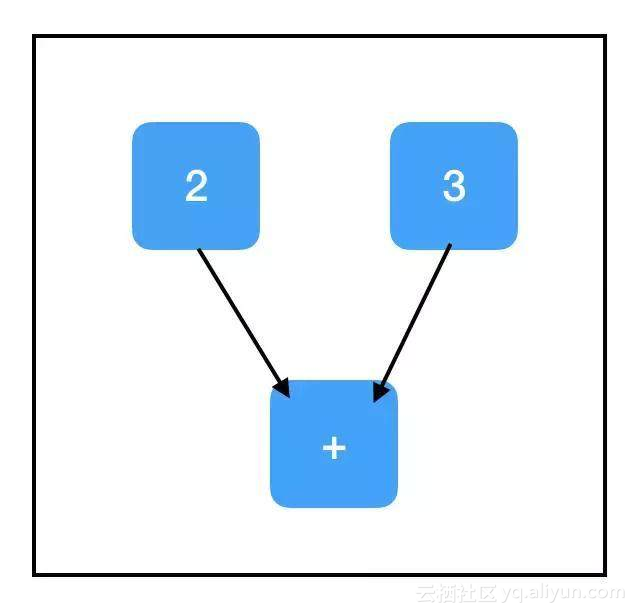import tensorflow as tftwo_node = tf.constant(2)three_node = tf.constant(3)sum_node = two_node + three_nodesess = tf.Session()print sess.run([two_node, sum_node])

[2, 5]import tensorflow as tfinput_placeholder = tf.placeholder(tf.int32)sess = tf.Session() print sess.run(input_placeholder)

Traceback (most recent call last):...InvalidArgumentError (see above for traceback): You must feed a value for placeholder tensor 'Placeholder' with dtype int32 [[Node: Placeholder = Placeholder[dtype=DT_INT32, shape=, _device="/job:localhost/replica:0/task:0/device:CPU:0"]()]]... 这是一个糟糕的例子，因为它引发了一个异常。占位符预计会被赋予一个值。但我们没有提供一个值，所以 TensorFlow 崩溃了。

import tensorflow as tf input_placeholder = tf.placeholder(tf.int32) sess = tf.Session() print sess.run(input_placeholder, feed_dict={input_placeholder: 2})

2import tensorflow as tfinput_placeholder = tf.placeholder(tf.int32)three_node = tf.constant(3)sum_node = input_placeholder + three_nodesess = tf.Session()print sess.run(three_node)print sess.run(sum_node)

3Traceback (most recent call last):...InvalidArgumentError (see above for traceback): You must feed a value for placeholder tensor 'Placeholder_2' with dtype int32 [[Node: Placeholder_2 = Placeholder[dtype=DT_INT32, shape=, _device="/job:localhost/replica:0/task:0/device:CPU:0"]()]]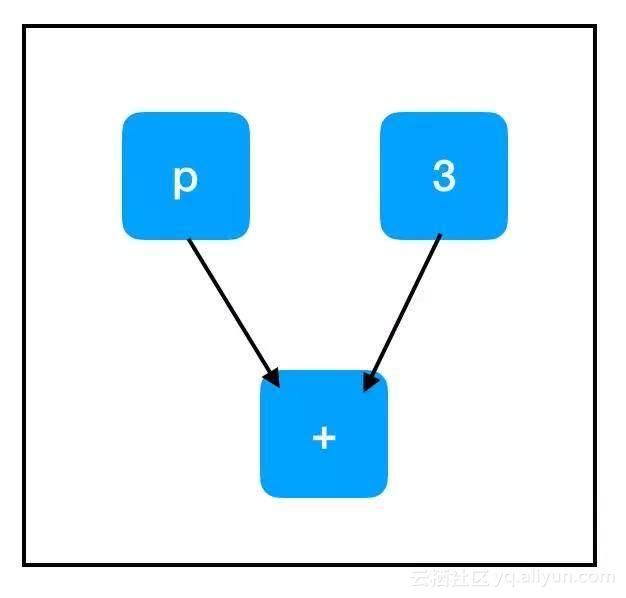sum_node 的计算路径：

TensorFlow 仅通过必需的节点自动进行计算这一事实是该框架的一个巨大优势。如果计算图非常大并且有许多不必要的节点，那么它可以节省大量调用的运行时间。它允许我们构建大型的「多用途」计算图，这些计算图使用单个共享的核心节点集合，并根据所采取的不同计算路径去做不同的事情。对于几乎所有应用而言，根据所采取的计算路径考虑 sess.run() 的调用是很重要的。

import tensorflow as tfcount_variable = tf.get_variable("count", [])sess = tf.Session()print sess.run(count_variable)

Traceback (most recent call last):...tensorflow.python.framework.errors_impl.FailedPreconditionError: Attempting to use uninitialized value count [Node: _retval_count_0_0 = _Retval[T=DT_FLOAT, index=0, _device="/job:localhost/replica:0/task:0/device:CPU:0"]]

import tensorflow as tfcount_variable = tf.get_variable("count", [])zero_node = tf.constant(0.)assign_node = tf.assign(count_variable, zero_node)sess = tf.Session()sess.run(assign_node)print sess.run(count_variable)

0

「副作用」节点支撑着大部分 Tensorflow 深度学习工作流程，所以请确保自己真正理解了在该节点发生的事情。当我们调用 sess.run(assign_node) 时，计算路径会通过 assign_node 和 zero_node。

import tensorflow as tfconst_init_node = tf.constant_initializer(0.)count_variable = tf.get_variable("count", [], initializer=const_init_node)sess = tf.Session()print sess.run([count_variable])

Traceback (most recent call last):...tensorflow.python.framework.errors_impl.FailedPreconditionError: Attempting to use uninitialized value count [Node: _retval_count_0_0 = _Retval[T=DT_FLOAT, index=0, _device="/job:localhost/replica:0/task:0/device:CPU:0"]]

import tensorflow as tfconst_init_node = tf.constant_initializer(0.)count_variable = tf.get_variable("count", [], initializer=const_init_node)init = tf.global_variables_initializer()sess = tf.Session()sess.run(init)print sess.run(count_variable)

0

1. 获取输入和 true_output
2. 根据输入和参数计算「推测」值
3. 根据推测与 true_output 之间的差异计算「损失」
4. 根据损失的梯度更新参数

import tensorflow as tf### build the graph## first set up the parametersm = tf.get_variable("m", [], initializer=tf.constant_initializer(0.))b = tf.get_variable("b", [], initializer=tf.constant_initializer(0.))init = tf.global_variables_initializer()## then set up the computationsinput_placeholder = tf.placeholder(tf.float32)output_placeholder = tf.placeholder(tf.float32)x = input_placeholdery = output_placeholdery_guess = m x + bloss = tf.square(y - y_guess)## finally, set up the optimizer and minimization nodeoptimizer = tf.train.GradientDescentOptimizer(1e-3)train_op = optimizer.minimize(loss)### start the sessionsess = tf.Session()sess.run(init)### perform the training loopimport random## set up problemtrue_m = random.random()true_b = random.random()for update_i in range(100000): ## (1) get the input and output input_data = random.random() output_data = true_m input_data + true_b ## (2), (3), and (4) all take place within a single call to sess.run()! loss, = sess.run([loss, train_op], feed_dict={input_placeholder: input_data, output_placeholder: output_data}) print update_i, _loss### finally, print out the values we learned for our two variablesprint "True parameters: m=%.4f, b=%.4f" % (true_m, true_b)print "Learned parameters: m=%.4f, b=%.4f" % tuple(sess.run([m, b]))

0 2.32053831 0.57927422 1.552543 1.57332594 0.64356485 2.40612656 1.07462567 2.19987158 1.67751169 1.646242310 2.441034...99990 2.9878322e-1299991 5.158629e-1199992 4.53646e-1199993 9.422685e-1299994 3.991829e-1199995 1.134115e-1199996 4.9467985e-1199997 1.3219648e-1199998 5.684342e-1499999 3.007017e-11True parameters: m=0.3519, b=0.3242Learned parameters: m=0.3519, b=0.3242

## finally, set up the optimizer and minimization nodeoptimizer = tf.train.GradientDescentOptimizer(1e-3)train_op = optimizer.minimize(loss)

train_op 回溯输入和损失的计算路径，寻找变量节点。对于它找到的每个变量节点，计算该变量对于损失的梯度。然后计算该变量的新值：当前值减去梯度乘以学习率的积。最后，它执行赋值操作更新变量的值。

import tensorflow as tftwo_node = tf.constant(2)three_node = tf.constant(3)sum_node = two_node + three_nodesess = tf.Session()print sess.run(sum_node)

5

import tensorflow as tftwo_node = tf.constant(2)three_node = tf.constant(3)sum_node = two_node + three_nodesess = tf.Session()answer, inspection = sess.run([sum_node, [two_node, three_node]])print inspectionprint answer

[2, 3]5

import tensorflow as tftwo_node = tf.constant(2)three_node = tf.constant(3)sum_node = two_node + three_nodeprint_sum_node = tf.Print(sum_node, [two_node, three_node])sess = tf.Session()print sess.run(print_sum_node)

25

import tensorflow as tftwo_node = tf.constant(2)three_node = tf.constant(3)sum_node = two_node + three_node### this new copy of two_node is not on the computation path, so nothing prints!print_two_node = tf.Print(two_node, [two_node, three_node, sum_node])sess = tf.Session() print sess.run(sum_node)

5

+ 关注